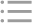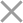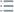## Fill out the form in 2 simple steps below:

Step 1

Step 2
** By clicking this button you agree to our Privacy Policy statementRequest our prices
--Select currency--
USD
EUR
* By clicking this button you agree to our Privacy Policy statementFree PVS‑Studio license for Microsoft MVP specialists
* By clicking this button you agree to our Privacy Policy statementTo get the licence for your open-source project, please fill out this form
* By clicking this button you agree to our Privacy Policy statementI am interested to try it on the platforms:
 Windows Linux macOS PVS-Studio for .NET Core JetBrains Rider
* By clicking this button you agree to our Privacy Policy statementMessage submitted.

Your message has been sent. We will email you at

check your Spam/Junk folder and click the "Not Spam" button for our message.
This way, you won't miss messages from our team in the future.

>
>
>
V1083. Signed integer overflow in arith…Introduction
Analyzing projects
On Windows
On Linux and macOS
Cross-platform
IDE
Build systems
Game Engines
Continuous use of the analyzer in software development
Deploying the analyzer in cloud Continuous Integration services
Managing analysis results
Analyzer diagnostics
General Analysis (C++)
General Analysis (C#)
General Analysis (Java)
Micro-Optimizations (C++)
Micro-Optimizations (C#)
Diagnosis of 64-bit errors (Viva64, C++)
Customer specific requests (C++)
MISRA errors
AUTOSAR errors
OWASP errors (C++)
OWASP errors (C#)
OWASP errors (Java)
Problems related to code analyzerContents

# V1083. Signed integer overflow in arithmetic expression. This leads to undefined behavior.

May 13 2022

The analyzer has detected an arithmetic expression in which a signed integer overflow may occur.

Example:

``````long long foo()
{
long longOperand = 0x7FFF'FFFF;
long long y = longOperand * 0xFFFF;
return y;
}``````

According to the C and C++ rules, the resulting type of the 'longOperand * 0xFFFF' expression will be 'long'. When you use the MSVC compiler on Windows, the size of 'long' type is 4 bytes. The maximum value that can be represented by this type is 2'147'483'647 in decimal or 0x7FFF'FFFF in hexadecimal. When multiplying the 'longOperand' variable by 0xFFFF (65,535), the 0x7FFF'7FFF'0001 result is expected. However, according to the C standard (see the C18 standard section 6.5 paragraph 5) and C++ (see standard C++20 section 7.1 paragraph 4), signed integer overflow leads to undefined behavior.

There are several ways to fix this code — it depends on the developer's intent.

If you need to make correct calculations, you need to use types whose sizes will be sufficient to fit a value. If the value does not fit a word, you can use one of the libraries for arbitrary-precision arithmetic. For example, GMP, MPRF, cnl.

The code fragment above can be corrected as follows:

``````long long foo()
{
long longOperand = 0x7FFF'FFFF;
long long y = static_cast<long long>(longOperand) * 0xFFFF;
return y;
}``````

If the signed integer overflow is an unexpected behavior, and it needs to be handled in some way, you can use special libraries to work with integers safely. For example, boost::safe_numerics or Google Integers.

If you need to implement wraparound arithmetic for signed integers with standard-defined behavior, you can use unsigned integers for calculations. In case of unsigned integer overflow, the integer is "wrapped" modulo '2 ^ n', where n is the number of bits of the integer.

Let's look at one of the possible solutions based on 'std::bit_cast' (C++20):

``````#include <concepts>
#include <type_traits>
#include <bit>
#include <functional>

namespace detail
{
template <std::signed_integral R,
std::signed_integral T1,
std::signed_integral T2,
std::invocable<std::make_unsigned_t<T1>,
std::make_unsigned_t<T2>> Fn>
R safe_signed_wrapper(T1 lhs, T2 rhs, Fn &&op)
noexcept(std::is_nothrow_invocable_v<Fn,
std::make_unsigned_t<T1>,
std::make_unsigned_t<T2>>)
{
auto uLhs = std::bit_cast<std::make_unsigned_t<T1>>(lhs);
auto uRhs = std::bit_cast<std::make_unsigned_t<T2>>(rhs);

auto res = std::invoke(std::forward<Fn>(op), uLhs, uRhs);

using UR = std::make_unsigned_t<R>;
return std::bit_cast<R>(static_cast<UR>(res));
}
}``````

The 'std::bit_cast' function converts 'lhs' and 'rhs' to the corresponding unsigned representations. Next, some arithmetic operation is performed on the two converted operands. Then the result expands or narrows to the needed resulting type and turns into a signed one.

With this approach, signed integers repeat the semantics of unsigned ones in arithmetic operations. This does not lead to undefined behavior.

For example, by clicking this link, you can see that the compiler may optimize the code if it detects that a signed integer overflow may occur. Let's take a closer look at the code fragment:

``````bool is_max_int(int32_t a)
{
return a + 1 < a;
}``````

If 'a' equals 'MAX_INT', the condition 'a + 1 < a' will be 'false'. This is a way to check whether an overflow has occurred. However, the compiler generates the following code:

``````is_max_int(int):                        # @is_max_int(int)
xor     eax, eax
ret``````

The assembly 'xor eax, eax' instruction resets the result of the 'is_max_int' function execution. As a result, the latter function always returns 'true', no matter what the value 'a' has. In this case, this is the result of undefined behavior due to overflow.

In the case of an unsigned representation, the undefined behavior does not happen:

``````is_max_int(int):                        # @is_max_int(int)
cmp     edi, 2147483647
sete    al
ret``````

The compiler has generated code that does check the condition.

This diagnostic is classified as:

 CWE-190CERT-INT32-C